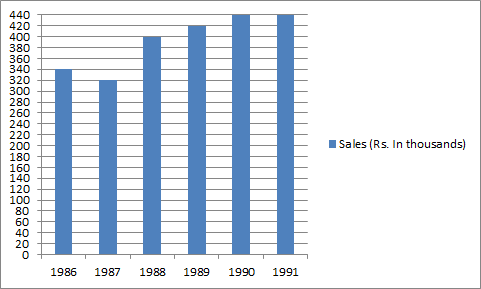# Data Interpretation - Bar Graph

>>>>>>>>Bar Graph

Instructions:

Study the following graph and answer the question 66-70   following it• A

Rs. 1000• B

Rs. 100000• C

Rs. 1000000• D

None of these• Option : B
• Explanation : (420-320) thousand = 100,000 i.e. one lakh

Instructions:

Study the following graph and answer the question 66-70   following it

• A

0.8• B

1.25• C

8• D

None of theseInstructions:

Study the following graph and answer the question 66-70   following it

• A

1986• B

1988• C

1989• D

1990• Option : D
• Explanation : Increased in 1989 over 1988 =  (20/400)*100 = 5

Increase in 1990 over 1989 = (20/400)*100 = 4.76

Instructions:

Study the following graph and answer the question 66-70   following it

• A

40• B

4• C

11• D

129.41• Option : D
• Explanation : 340*X = 440 or X = (440*100)/340 = 129.41%

Instructions:

Study the following graph and answer the question 66-70   following it

• A

420• B

425• C

430• D

None of these• Option : D
• Explanation : (400+420+440+440)/4 = 425
Related Quiz.
Bar Graph